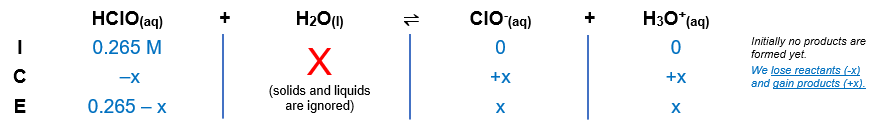# Problem: Determine the [H3O+] in a 0.265 M HCIO solution. The Ka of HCIO is 2.9 x 10-8.A) 1.1 × 10-10 M B) 8.8 × 10-5 M C) 4.9 × 10-4 M D) 7.7 × 10-9 M E) 1.3 × 10-6 M

###### FREE Expert Solution

Equilibrium reaction:        HClO(aq) + H2O(l)  ClO-(aq) + H3O+(aq)

Step 1: Construct an ICE chart for the reaction.Step 2: Write the Ka expression and calculate for Ka.

$\overline{){{\mathbf{K}}}_{{\mathbf{a}}}{\mathbf{=}}\frac{\mathbf{products}}{\mathbf{reactants}}}\phantom{\rule{0ex}{0ex}}{\mathbf{K}}_{\mathbf{a}}\mathbf{=}\frac{\mathbf{\left[}{\mathbf{ClO}}^{\mathbf{-}}\mathbf{\right]}\mathbf{\left[}{\mathbf{H}}_{\mathbf{3}}{\mathbf{O}}^{\mathbf{+}}\mathbf{\right]}}{\left[\mathrm{HClO}\right]}$

Solids and liquids are not included in the expression

Step 3: Calculate the equilibrium concentrations.

${\mathbf{K}}_{\mathbf{a}}\mathbf{=}\frac{\left[\mathbf{x}\right]\left[\mathbf{x}\right]}{\left[\mathbf{0}\mathbf{.}\mathbf{265}\mathbf{-}\mathbf{x}\right]}\phantom{\rule{0ex}{0ex}}\mathbf{2}\mathbf{.}\mathbf{9}\mathbf{×}{\mathbf{10}}^{\mathbf{-}\mathbf{8}}\mathbf{=}\frac{{\mathbf{x}}^{\mathbf{2}}}{\mathbf{0}\mathbf{.}\mathbf{265}\mathbf{-}\mathbf{x}}$

98% (114 ratings)###### Problem Details

Determine the [H3O+] in a 0.265 M HCIO solution. The Ka of HCIO is 2.9 x 10-8.

A) 1.1 × 10-10

B) 8.8 × 10-5

C) 4.9 × 10-4

D) 7.7 × 10-9

E) 1.3 × 10-6 M